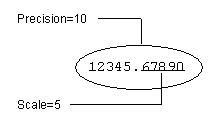2. SQL Data Types : Data Types : Decimal Data Type

Decimal Data Type
The decimal data type is an exact numeric data type defined by its precision (total number of digits) and scale (number of digits to the right of the decimal point). For example:Note:  The decimal data type is suitable for storing currency data where the required range of values or number of digits to the right of the decimal point exceeds the capacities of the money data type. For display purposes, a currency sign cannot be specified for decimal values.
Specify the decimal data type using the following syntax:
decimal(p,s)
where:
p
Defines the precision. Minimum is 1; maximum is 38.
s
Defines the scale. The scale of a decimal value cannot exceed its precision. Scale can be 0 (no digits to the right of the decimal point).
Note:  Synonyms for the decimal data type are dec and numeric.
The following defines a type with precision of 23 decimal digits, 5 of which are after the decimal point:
DECIMAL(23,5)